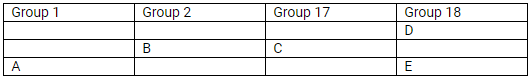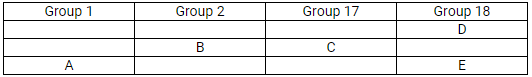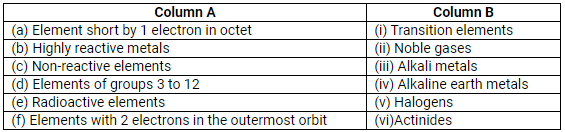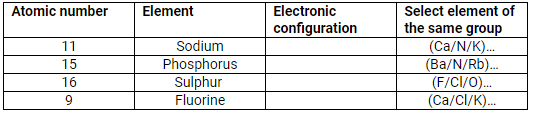## Selina Concise Chemistry Class 9 ICSE Solutions The Periodic Table

APlusTopper.com provides step by step solutions for Selina Concise ICSE Solutions for Class 9 Chemistry Chapter 5 The Periodic Table. You can download the Selina Concise Chemistry ICSE Solutions for Class 9 with Free PDF download option. Selina Publishers Concise Chemistry for Class 9 ICSE Solutions all questions are solved and explained by expert teachers as per ICSE board guidelines.

Selina ICSE Solutions for Class 9 Chemistry Chapter 8 The Periodic Table

Page No. 79

Question 1.
What is the need for classification of elements?
Solution:
It is impossible for a chemist to study all the elements and their compounds. Hence, classification is a must.
Following are the reasons for the classification of elements:

1. To study elements better
2. To correlate the properties of the elements with some fundamental properties which are characteristic of all the elements
3. To reveal relationships between elements

Question 2.
What was the basis of the earliest attempts made for classification and grouping of elements?
Solution:
The first classification of elements was into 2 groups-metals and non-metals.

Question 3(a).
A, B and C are the elements of a Dobereiner’s triad. If the atomic mass of A is 7 and that of C is 39, what should be the atomic
mass of B?
Solution:

(a)Question 3(a).
Solution:
(b) Döbereiner failed to arrange all the known elements in the form of triads.
In the triad of fluorine (19), chlorine (35.5) and bromine (80), it is observed that the mean of the atomic masses of fluorine and bromine is ½(19 + 80) = 49.5, not 35.5.

Question 4.
Explain ‘Newland’s Law of Octaves.’ Why was the law discarded?
Solution:
Elements when arranged in the increasing order of their atomic weights are similar to the eighth and the first note of the musical scale. For example, the eighth element from lithium is sodium. Similarly, the eighth element from sodium is potassium. Thus, lithium and sodium provide any specific place for hydrogen.

1. This classification did not work with heavier elements.
2. Newland adjusted two elements Cobalt (Co) and Nickel (Ni) in the same slot.
3. Fe, which resembles Co and Ni in properties, has been placed far away.

Question 5.
Did Dobereiners triads also exist in the columns of Newland’s Octaves? Compare and find out.
Solution:
Yes, Döbereiner’s triads also exist in the columns of Newland’s octaves. For example, the second column of Newlands classification has the elements Lithium (Li), Sodium (Na) and Potassium (K), which constitute a Döbereiner’s triad.

Question 6(a).
Lithium, sodium and potassium elements were put in one group on the basis of their similar properties. What are those similar properties?
Solution:
(a) Elements of lithium, sodium and potassium have the following similar properties:

1. All these have one electron in the outermost shell.
2. They form unipositive ions.
3. They are good reducing agents.
4. They are soft metals.
5. They impart colour to the flame.
6. Common name of the group is alkali metals [Group 1A].

Question 6(b).
The elements calcium, strontium and barium were put in one group or family on the basis of their similar properties.
What were those similar properties?
Solution:
(b)

1. All of them are metals.
2. Oxide of each of them is alkaline in nature.
3. Each has valency 2.

Question 7(a).
What was Mendeleev’s basis for classification of elements?
Solution:
(a) Mendeleev’s basis for periodic classification:

1. Similarities in the chemical properties of elements.
2. Increasing order of atomic weights of elements.

Question 7(b).
Mendeleev’s contributions to the concept of periodic table laid the foundation for the Modern Periodic Table. Give reasons.
Solution:
(b) Mendeleev laid the foundation for the modern periodic table by showing periodicity of the properties of the elements by arranging the elements (63) then known into 8 groups, by leaving gaps for undiscovered elements and predicting their properties. He made separate groups for metals and non-metals. He also created periods in which the element gradually changes from metallic to non-metallic character. He was also able to show that the element in the same sub-group had the same valency.

Question 8.
State Mendeleev’s periodic law.
Solution:
Mendeleev’s periodic law: The physical and chemical properties of all the elements are a periodic function of their atomic masses.

Question 9(a).
Use Mendeleev’s Periodic Table to predict the formula of hydrides of carbon and silicon.
Solution:
(a) C is in Group 4. So, the hydride will be CH4 (Methane).
Si is in Group 4. So, the hydride will be SiH4 (Silane).

Question 9(b).
Use Mendeleev’s Periodic Table to predict the formula of oxides of potassium, aluminium and barium.
Solution:
(b)
K is in Group 1. So, the oxide will be K2O (Potassium oxide).
Al is in Group 3. So, the oxide will be Al2O3 (Aluminium oxide).
Ba is in Group 2. So, the oxide will be BaO (Barium oxide).

Question 10.
Which group of elements was missing from Mendeleev’s original periodic table?
Solution:
Anomalous pairs of elements were missing from Mendeleev’s periodic table.

Question 11.
State the merits of Mendeleev’s classification of elements.
Solution:
Merits of Mendeleev’s classification of elements:

1. Grouping of elements
2. Gaps for undiscovered elements: Mendeleev left some gaps in his periodic table for subsequent inclusion of elements not known at that time.
3. He predicted the properties of the then unknown elements on the basis of properties of elements lying adjacent to the vacant slots (eka-aluminium and eka-silicon).

Question 12.
Why did Mendeleev’s leave some gaps in his periodic table os elements? Explain your answer with an example.
Solution:
He left gaps in the table for the undiscovered elements. He discovered the properties of such elements with the help of neighboring elements.
He discovered eka-silicon with atomic mass of 72 which was later named Germanium with atomic mass 72.6.

Question 13.
The atomic number of an element is more important to the chemist than its relative atomic mass. Why?
Solution:
Henry Moseley found that when cathode rays struck anodes of different metals, the wavelength of these metals was found to decrease in a regular manner of changing the metal of anode in the order of its position in the periodic table. By this, he concluded that the number of positive charges present in the nucleus due to protons (atomic number) is the most fundamental property of the element.

So, Henry Moseley found that the atomic number is a better fundamental property of an element compared to its atomic mass. This lead to the modern periodic law.

This law gave explanations for anomalies in Mendeleev’s classification of elements such as

1. Position of isotopes with the same atomic number can be put in one place in the same group.
2. Position of argon and potassium: Potassium with higher atomic number should come later, and argon with lower atomic number should come first.

Question 14.
Consider the following elements: Be, Li, Na, Ca, K. Name the elements of (a) same group (b) same period.
Solution:

 Element At. No. Electronic distribution Be 4 2, 2 Li 3 2, 1 Na 11 2, 8, 1 Ca 20 2, 8, 8 K 19 2, 8, 8, 2
1. Same IA group (Li, Na, K) and IIA group (Be, Ca)
2. In the second period (Be, Li) and in the fourth period (K, Ca)

Question 15(a).
Name an element whose properties were predicted on the basis of its position in Mendeleev’s periodic table.
Solution:
a. Eka-silicon

Question 15(b).
Name two elements whose atomic weights were corrected on the basis of their positions in Mendeleev’s periodic table.}
Solution:
b. Gold and Platinum

Question 15(c).
How many elements were known at the time of Mendeleev’s classification of elements?
Solution:
c. Only 63 elements were discovered at the time of Mendeleev’s classification of elements.

Page No. 86

Question 1(a).
State the modern periodic law.
Solution:
a. Modern periodic law: The physical and chemical properties of all elements are a periodic function of their atomic numbers.

Question 1(b).
How many periods and groups are there in the modern periodic table?
Solution:
b. Eighteen groups and seven periods

Question 2.
What is the main characteristic of the last elements in the periods of a periodic table? What is the general name of such elements?
Solution:
Last elements of each period have their outermost shell complete, i.e. 2 or 8 electrons.
The general name is inert gases or noble gases.

Question 3(a).
What is meant in the periodic table by a group?
Solution:
a. Vertical columns in a periodic table which have the same number of valence electrons and similar chemical properties are called a group.

Question 3(b).
What is meant in the periodic table by a period?
Solution:
b. In a periodic table, elements are arranged in the order of increasing atomic numbers in horizontal rows called periods.

Question 4.
From the standpoint of atomic structure, what determines which elements will be the first and which the last in a period of the periodic table?
Solution:
Atomic number determines which element will be the first and which will be the last in a period of the periodic table.

Question 5(a).
What are the following groups known as?

1. Group 1
2. Group 17
3. Group 18

Solution:

(a)

1. Group 1 is known as the alkali metals.
2. Group 17 is known as the halogens.
3. Group 18 is known as the transition elements.

Question 5(b).
Name two elements of each group.
Solution:
(b)

1. Group 1: Lithium (Li), Sodium (Na)
2. Group 17: Chlorine (Cl), Iodine (I)
3. Group 18: Helium (He), Neon (Ne)

Question 6(a).
What is the number of elements in the 1st period?
Solution:
(a) There are two elements in the first period.

Question 6(b).
What is the number of elements in the 3rd period, of the modern periodic table?
Solution:
(b) There are eight elements in the third period.

Question 7(a).
How does number of (i) valence electrons (ii) valency; vary on moving from left to right in the second period of a periodic table?
Solution:

(a)

1. The valence electrons in the same shell (outermost shell) increase progressively by one across the period. The first element hydrogen has one valence electron and helium has two valence electrons.
2. On moving from left to right in a period, valency increases from 1 to 4, then falls to one and ultimately to zero in the last group.

Question 7(b).
How does number of (i) valence electrons (ii) valency; vary on moving from left to right in the third period of a periodic table?
Solution:

(b)

1. Valence electrons in the same shell (outermost shell) increase progressively by one across the period. The first element sodium has one valence electron and magnesium has two valence electrons.
2. On moving from left to right in the third period, valency increases from 1 to 7 and ultimately to zero in the last group.

Question 8.
How do atomic structures (electron arrangements) change in a period with increase in atomic numbers moving left to right?
Solution:
The size of atoms decreases when moving from left to right in a period. Thus, in a particular period, the alkali metal atoms are the largest and the halogen atoms are the smallest.

Question 9(a).
This question refers to elements of the periodic table with atomic numbers from 3 to 18. In the table below, some elements are shown by letters, even though the letters are not the usual symbols of the elements.Which of these is:

1. a noble gas?
2. a halogen?
3. an alkali metal?
4. an element with valency 4?

Solution:

(a)

1. H and P are noble gases.
2. G and O are halogens.
3. A and I are alkali metals.
4. D and L have valency 4.

Question 9(b).
This question refers to elements of the periodic table with atomic numbers from 3 to 18. In the table below, some elements are shown by letters, even though the letters are not the usual symbols of the elements.If A combines with F, what would be the formula of the resulting compound?
Solution:
(b) Li2O. A stands for lithium and F stands for oxygen. The valence of lithium is +1 and the valence of O is -2, i.e. A2F.

Question 9(c).
This question refers to elements of the periodic table with atomic numbers from 3 to 18. In the table below, some elements are shown by letters, even though the letters are not the usual symbols of the elements.Solution:
(c) G has atomic number 9; therefore, its electronic arrangement is 2, 7.

Question 10.
Sodium and aluminium have atomic numbers 11 and 13, respectively. They are separated by one element in the periodic table, and have valencies 1 and 3 respectively. Chlorine and potassium are also separated by one element in the periodic table (their atomic numbers being 17 and 19, respectively) and yet both have valency 1. Explain.
Solution:
Na and Al have the capacity to donate an electron due to which the valency is positive, whereas Cl and K can only gain or lose one electron due to which their valency is -1 and +1, respectively. This is the only difference between these two.

Question 11.
Helium is an unreactive gas and neon is a gas of extremely low reactivity. What, if anything, do their atoms have in common.
Solution:
These elements have a full outermost subshell, which results in high stability. They only react with other elements in extreme circumstances.

Question 12(a).
In which part of a group would you separately expect the elements to have the greatest metallic character?
Solution:
a. The greatest metallic character can be expected at the bottom of the group.

Question 12(b).
In which part of a group would you separately expect the elements to have the largest atomic size?
Solution:
b. The largest atomic size can be expected at the lower part of the group.

Question 13.
What happens to the number of valence electrons in atoms of elements as we go down a group of the periodic table?
Solution:
The number of valence electrons remains the same as we go down a group.

Question 14(a).
The position of elements A, B, C, D and E in the periodic table are shown below:State which are metals, non-metals and noble gas in this table.
Solution:
(a) Metals: A and B; Non-metals: C; Noble gases: D and E

Question 14(b).
The position of elements A, B, C, D and E in the periodic table are shown below:State which is most reactive (i) metal (ii) non-metal
Solution:
(b) Most reactive

1. Metals: Alkali metals (Group I); Caesium
2. Non-metals: Halogens (Group 17); Fluorine

Question 14(c).
The position of elements A, B, C, D and E in the periodic table are shown below:Which type of ion will be formed by element A, B and C.
Solution:

(c)

Element A will form a positive ion 1+ (cation).
Element B will form a positive ion 2+ (cation).
Element C will form a negative ion 1 (anion).

Question 14(d).
The position of elements A, B, C, D and E in the periodic table are shown below:Which is larger in size (i) D or E (ii) B or C
Solution:
(d)

1. E
2. B

Question 15.
Write the electronic configuration of element 17T35.

1. What is the group number of T?
2. What is the period number of T?
3. How many valence electrons are there in an atom of T?
4. What is the valency of T?
5. Is it a metal or non-metal?
6. State number of protons and neutrons in T.

Solution:
K L M
Electronic configuration = 2, 8, 7

a. VIIA
b. Third period
c. Seven
d. Valency of T = -1
e. Non-metal
f. Protons = 17, Neutrons = 18

Page No. 91

Question 1.
Element P has atomic number 19. To which group and period, does P belong? Is it a metal or a non-metal? Why?
Solution:
Atomic number of P = 19
Electronic configuration = 2, 8, 8, 1
Group number of the element = 1
A Period number of the element = 4
P is a metal.

Question 2.
An element belongs to the third period and Group IIIA (13) of the periodic table. State:
a. the number of valence electrons,
b. the valency,
c. if it is a metal or non-metal?
d. the name of the element.
Solution:
a. 3
b. +3
c. Metal
d. Aluminium

Question 3.
Name and state the following with reference to the elements of the first three periods of the periodic table.

1. Noble gas with duplet arrangement of electrons.
2. Metalloid in Period 3.
3. Valency of elements in Group 14 and 15.
4. Noble gas having electronic configuration: 2, 8, 8.
5. Group whose elements have zero valency.
6. A covalent compound formed by an element in period 2 and ahalogen.
7. Non-metallic element present in Period 3 of Groups 15 and 16
8. An electrovalent compound formed by an alkaline earth metal and a halogen.
9. Bridge elements of Period 3 of Group 1,2 and 3.
10. Alkali metal in period 3 that dissolves in water giving a strong alkali.
11. Typical elements of Groups 14 and 15.
12. Alkaline earth metal in period 3.

Solution:

(a) Helium
(b) Silicon
(c) 4, 3
(d) Argon
(e) Noble gases
(f) Carbon tetrachloride (CCl4)
(g) Silicon, Phosphorus
(h) Sodium chloride (Na+Cl)
(i) Li and Mg; Be and Al; B and Si
(j) Sodium
(k) Typical elements of Period 2 belonging to group 14 and 15 are carbon and nitrogen.
Typical elements of’ Period 3 belonging to group 14 to 15 are silicon and phosphorus.
(l) Beryllium

Question 4.
Match column A with column BSolution:

 Column A Column B (a) Elements short of 1 electron in octet (v) Halogens (b) Highly reactive metals (iii) Alkali metals (c) Unreactive elements (ii) Noble gases (d) Elements of groups 3 to 12 (i) Transition elements (e) Radioactive elements (vi) Actinides (f) Elements with 2 electrons in outermost orbit (iv) Alkaline earth metals

Question 5.
Complete the table:Solution:

 Atomic number Element Electronic configuration Select element of the same group 11 Sodium 2, 8, 1 K 15 Phosphorus 2, 8, 5 N 16 Sulphur 2, 8, 6 O 9 Fluorine 2, 7 Cl

Question 6.

1. Write down the word that will correctly complete the following sentences:
2. Relative atomic mass of a light element up to calcium is approximately_____ its atomic number.
3. The horizontal rows in a periodic table are called _____ .
4. Going across a period left to right, atomic size _____ .
5. Moving left to right in the second period, number of valence electrons _____ .
6. Moving down in the second group, number of valence electrons___________.

Solution:

a. Relative atomic mass of a light element up to calcium is approximately 20 its atomic number.
b. The horizontal rows in a periodic table are called periods.
c. Going across a period left to right, atomic size increases.
d. Moving left to right in the second period, number of valence electrons increases from 1 to 8.
e. Moving down in the second group, number of valence electrons remain same.

Question 7(a).
Name the alkali metals, How many electron(s) they have in their outermost orbit.
Solution:
Name of the alkali metals: Lithium, sodium, potassium, rubidium, cesium and francium
Electrons in the outermost orbit: 1

Question 7(b).
Take any one alkali metal and write its reaction with (i)oxygen (ii)water (iii)acid.
Solution:

1. Reaction of alkali metal with oxygen – React rapidly with oxygen
4Na + O2 → 2Na2O
2. Reaction of alkali metal with water – React with water violently and produce hydrogen
2M + 2H2O → 2MOH + H2
3. Reaction of alkali metal with acid – React violently with dil. HCl and dil. H2SO4 to produce hydrogen
2M + 2HCl → 2MCl + H2

Page No. 92

Question 8(a).
Name the method by which alkali metals can be extracted.
Solution:
a. Alkali metals can be extracted by the electrolysis of their molten salts.

Question 8(b).
What is the colour of the flame of sodium and potassium?
Solution:
b. The colour of the flame of sodium is golden yellow, and the colour of the flame of potassium is pale violet.

Question 9(a)
Name the first three alkaline earth metals.
Solution:
a. The first three alkaline earth metals are Beryllium, Magnesium and Calcium.

Question 9(b)
Write the reactions of first three alkaline earth metals with dilute hydrochloric acid.
Solution:
b. Reactions of the first three alkaline earth metals with dilute hydrochloric acid:
Be + 2HCl → BeCl2 + H2
Mg + 2HCl → MgCl2 + H2
Ca + 2HCl → CaCl2 + H2

Question 10(a)
How do alkaline earth metals occur in nature?
Solution:
a. Alkaline earth metals occur in nature in the combined state and not in the free state as they are very reactive.

Question 10(b)
Write the electronic configuration of the first two alkaline earth metals.
Solution:
b. Electronic configuration of the first two alkaline earth metals:
4Be: 1s22s2
12Mg: 1s22s22p63s2

Solution 11.
a. Group 17 elements are called halogens. The name halogens is from Greek halo (sea salt) and gens (producing, forming) and thus means ‘sea salt former’.

b. Group 17 elements or halogens:

1. Reactivity: Halogens are the most reactive non-metals, their reactivity decreases down the group. Fluorine is the most reactive halogen and iodine is the least reactive halogen.
2. Colour: Fluorine is a pale yellow gas, chlorine is a greenish yellow gas, bromine is a reddish brown liquid and iodine is a violet solid.
3. Physical state: Gaseous

Solution 12.
(a)

1. Group 17 elements react with metals to form metal halides which are neutral in nature.
2. Group 17 elements react with non-metals to form acidic compounds such as hydrogen halides.

(b) Group 17 elements are highly reactive because of their closeness to the noble or stable gas configuration. They can easily achieve a noble gas electron structure.

Solution 13.
a. All the noble or inert gases have 8 electrons in their valence shell except helium which has two electrons in its valence shell.
b. Xenon or krypton from Group 18 can form compounds.

Solution 14.

1. Helium gas is used in airships and balloons.
2. Neon gas is used in neon lights. The brightly coloured advertising light works when an electric discharge is passed in a tube containing a little of a noble gas such as neon.

Question 9.
An element A has 2 electrons in its fourth shell. State:

1. its atomic number
2. its electronic configuration
3. its valency
4. position in the periodic table
5. is it a metal or non metal
6. is it an oxidising or reducing agent

Solution 15.

a. Ca
b. 1s22s22p63s23p64s2
c. 2
d. Group 2 Period 4
e. Metal
f. Reducing agent

More Resources for Selina Concise Class 9 ICSE Solutions